## Example Questions

### Example Question #11 : Plane Geometry

"O" is the center of the circle as shown below.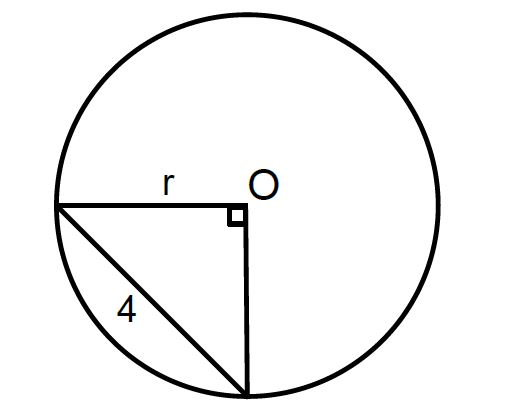A

---

B

---

3

The relationship cannot be determined

Quantity B is greater

The two quantities are equal

Quanitity A is greater

Quantity B is greater

Explanation:

We know the triangle inscribed within the circle must be isosceles, as it contains a 90-degree angle and fixed radii. As such, the opposite angles must be equal. Therefore we can use a simplified version of the Pythagorean Theorem,

a2 + a= c2 → 2r= 16 → r2 = 8; r = √8 < 3. (since we know √9 = 3, we know √8 must be less); therefore, Quantity B is greater.

### Example Question #1 : How To Find The Length Of A Radius

Which point could lie on the circle with radius 5 and center (1,2)?

(–3, 6)

(3,4)

(3,–2)

(4,–1)

(4,6)

(4,6)

Explanation:

A radius of 5 means we need a distance of 5 from the center to any points on the circle. We need 52 = (1 – x2)2 + (2 – y2)2. Let's start with the first point, (3,4). (1 – 3)2 + (2 – 4)≠ 25. Next let's try (4,6). (1 – 4)2 + (2 – 6)2 = 25, so (4,6) is our answer. The same can be done for the other three points to prove they are incorrect answers, but this is something to do ONLY if you have enough time.

### Example Question #1 : How To Find The Length Of A Radius

A circular fence around a monument has a circumference of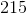feet. What is the radius of this fence?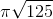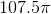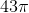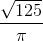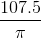Explanation:

This question is easy on the whole, though you must not be intimidated by one small fact that we will soon see. Set up your standard circumference equation:The circumference isfeet, so we can say: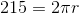Solving for, we get: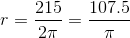Some students may be intimidated by havingin the denominator; however, there is no need for such intimidation. This is simply the answer!

### Example Question #2 : How To Find The Length Of A Radius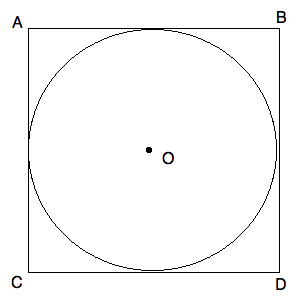Circle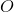has a center in the center of Square.

The area of Square ABCD is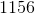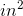.

What is the radius of Circle?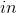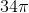Explanation:

Since we know that the area of Squareis, we know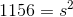, whereis the length of one of its sides. From this, we can solve forby taking the square root of both sides. You will have to do this by estimating upward. Therefore, you know that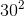is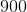. By careful guessing, you can quickly see that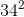is. From this, you know that the diameter of your circle must be half of, or(because it is circumscribed). Therefore, you can draw: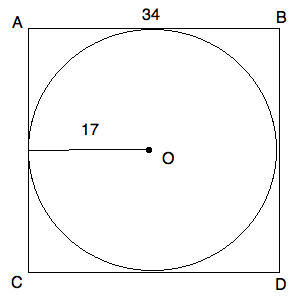Tired of practice problems?

Try live online GRE Math prep today.